# Contents Finding half Finding quarter Finding one third

• Slides: 16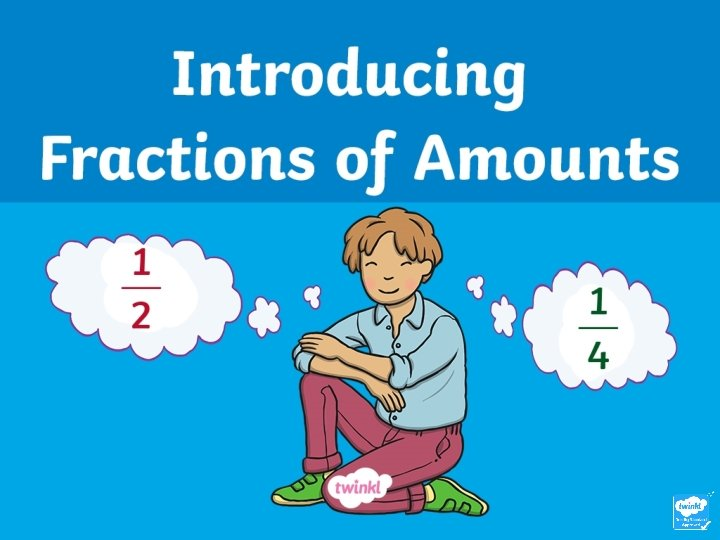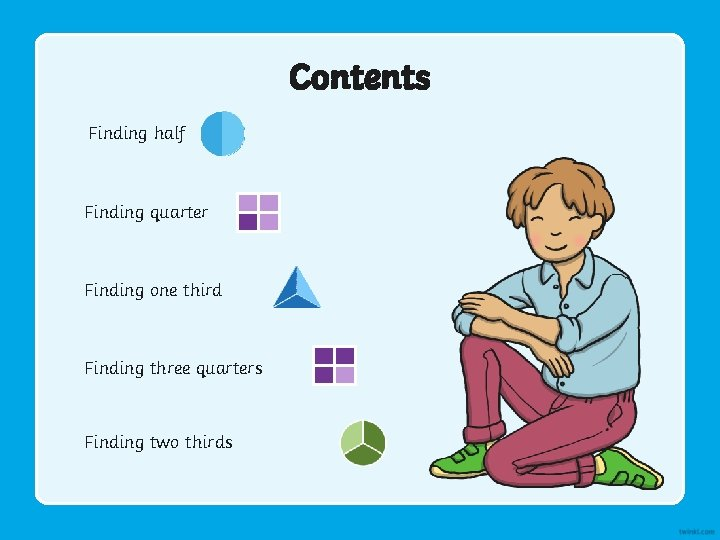Contents Finding half Finding quarter Finding one third Finding three quarters Finding two thirdsFinding Half (½) To find half of a number of objects, you need to divide them by two. Count the number of cakes. There are 8 cakes.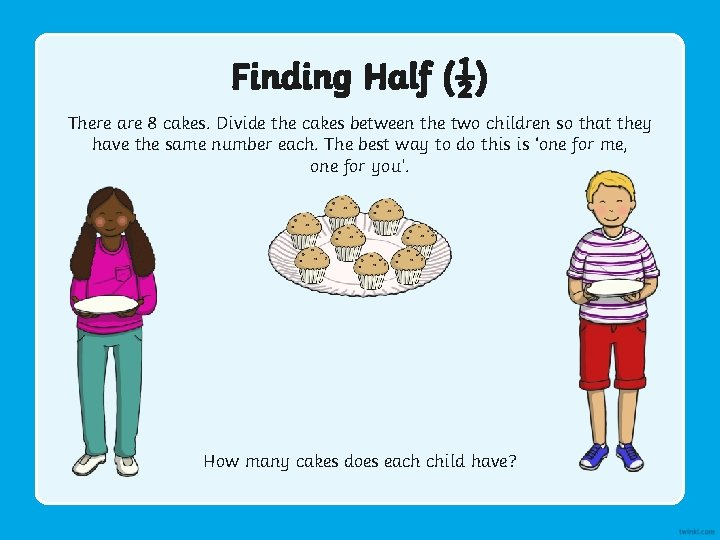Finding Half (½) There are 8 cakes. Divide the cakes between the two children so that they have the same number each. The best way to do this is ‘one for me, one for you’. How many cakes does each child have?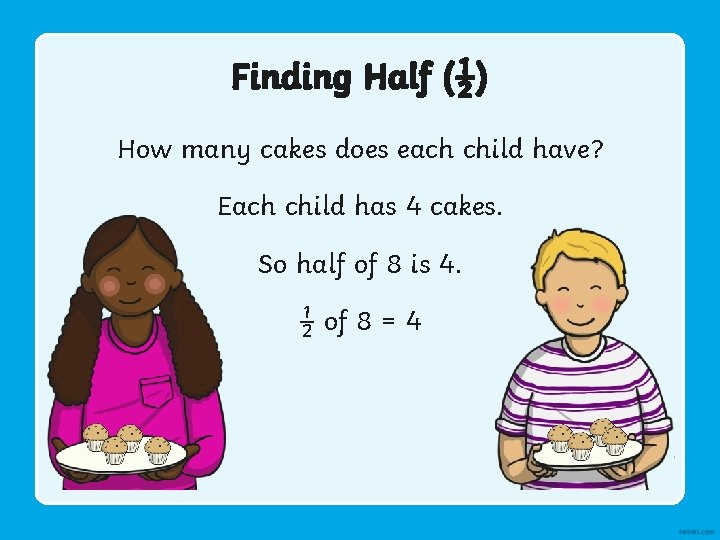Finding Half (½) How many cakes does each child have? Each child has 4 cakes. So half of 8 is 4. ½ of 8 = 4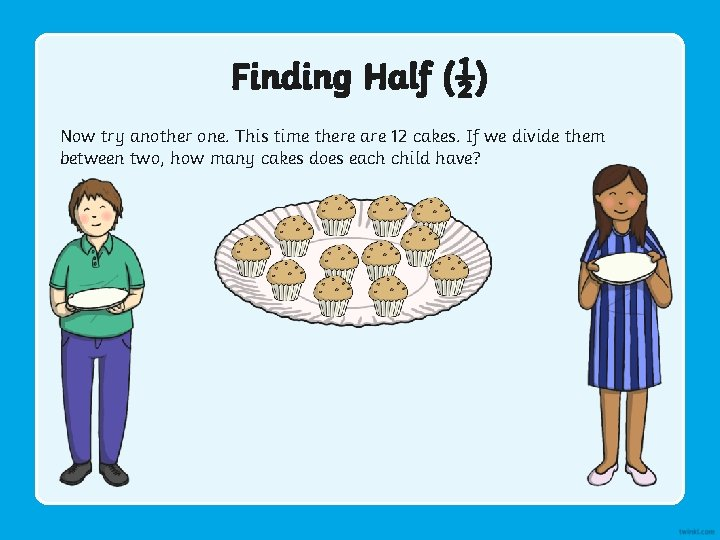Finding Half (½) Now try another one. This time there are 12 cakes. If we divide them between two, how many cakes does each child have?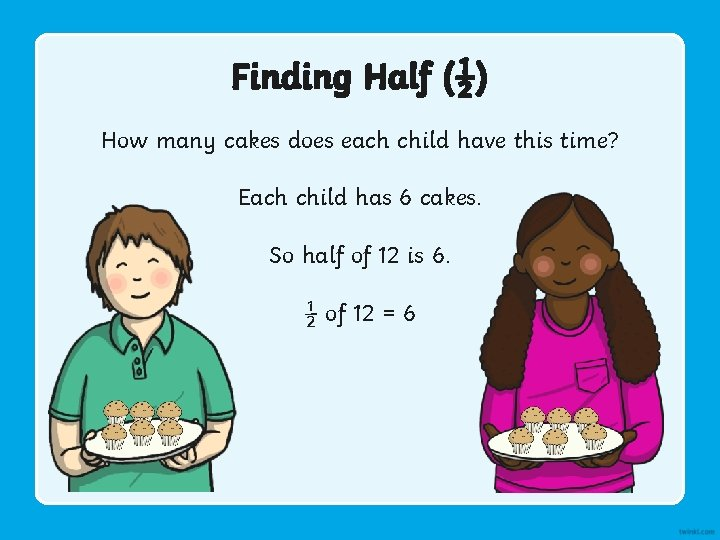Finding Half (½) How many cakes does each child have this time? Each child has 6 cakes. So half of 12 is 6. ½ of 12 = 6Finding a Quarter (¼) You need to divide by 4 to find one quarter. Count the number of bones.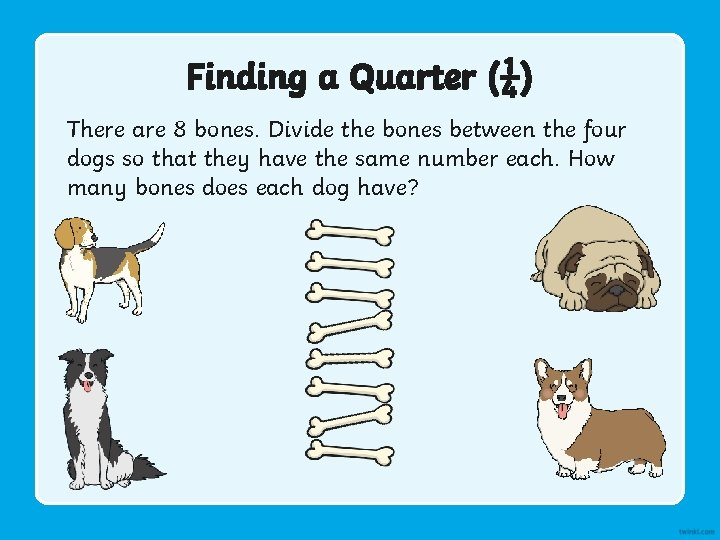Finding a Quarter (¼) There are 8 bones. Divide the bones between the four dogs so that they have the same number each. How many bones does each dog have?Finding a Quarter (¼) How many bones does each dog have? Each dog has 2 bones. So quarter of 8 is 2. ¼ of 8 = 2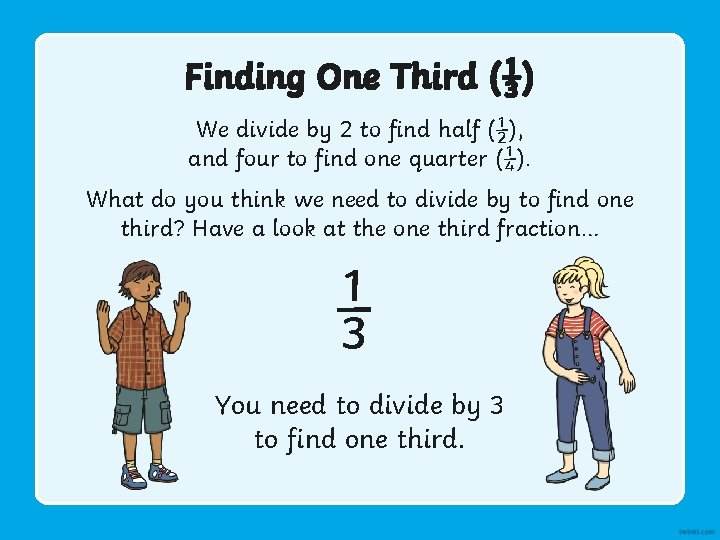Finding One Third (⅓) We divide by 2 to find half (½), and four to find one quarter (¼). What do you think we need to divide by to find one third? Have a look at the one third fraction… ⅓ You need to divide by 3 to find one third.Finding One Third (⅓) Here are 9 butterflies. Divide them between the three flowers so that the same number is on each flower. How many butterflies are on each flower?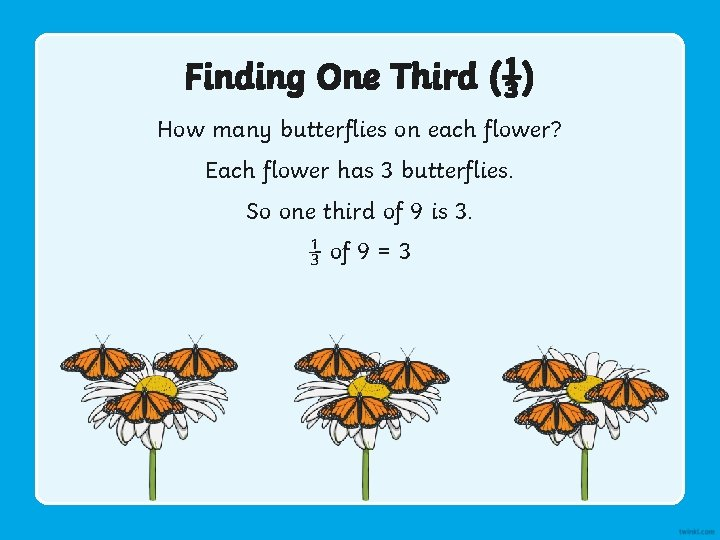Finding One Third (⅓) How many butterflies on each flower? Each flower has 3 butterflies. So one third of 9 is 3. ⅓ of 9 = 3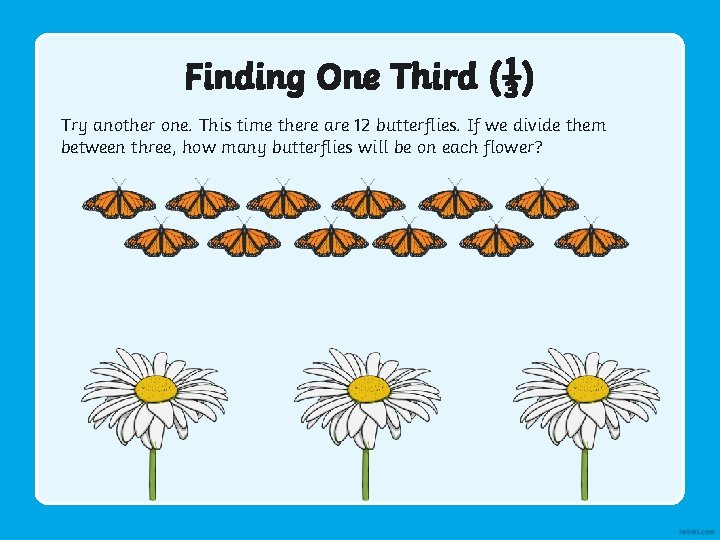Finding One Third (⅓) Try another one. This time there are 12 butterflies. If we divide them between three, how many butterflies will be on each flower?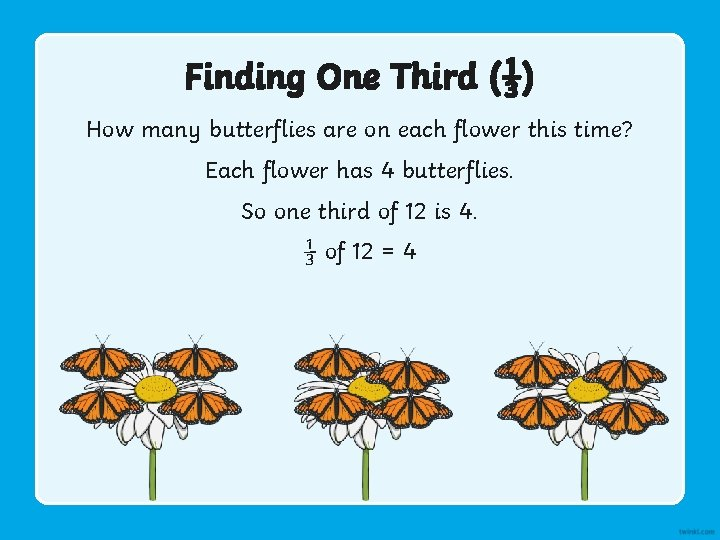Finding One Third (⅓) How many butterflies are on each flower this time? Each flower has 4 butterflies. So one third of 12 is 4. ⅓ of 12 = 4